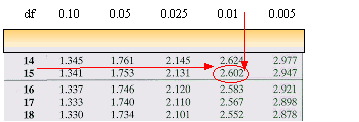### CFA Practice Question

There are 985 practice questions for this topic.

### CFA Practice Question

For the t-distribution, if a = 0.01 and n = 16, the t-value is ______.

A. 2.583
B. 2.602
C. 1.341

Reading the t-table, we go to column 0.01 then down to row 16 - 1 or row 15. The value is 2.602.User Comment
SuperKnight Do we have to memorize t-table values as well?
kenchew1 Even without the table, in this case, a=0.01 => 99% confidence level. For a z-statistic, the z-value = 2.58. Since this is t-statistics, recall that its bell-curve has a lower peak and fatter tails, so for it to have the same 99% confidence level, the t-value>2.58. There is only one answer > 2.58, so the answer is B.
thekid CONFUSED...

Shouldn't we look at a/2 = 0.01/2 = 0.005 Since it is 99% C.I. that would mean 0.5% lies on each end (left & right side) of the interval...?